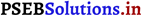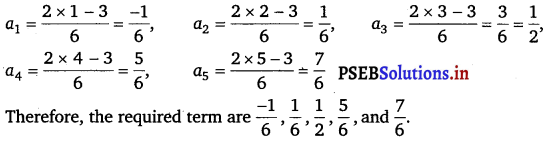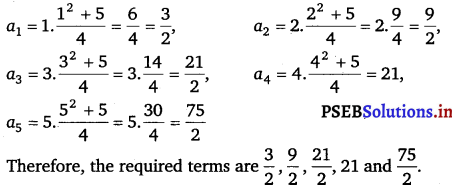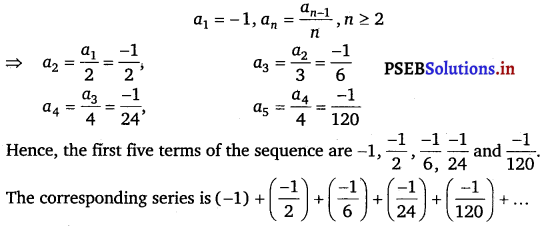# PSEB 11th Class Maths Solutions Chapter 9 Sequences and Series Ex 9.1

Punjab State Board PSEB 11th Class Maths Book Solutions Chapter 9 Sequences and Series Ex 9.1 Textbook Exercise Questions and Answers.

## PSEB Solutions for Class 11 Maths Chapter 9 Sequences and Series Ex 9.1

Question 1.
Write the first five terms of the sequence whose nth term is an = n (n + 2).
an = n(n +2)
Substituting n = 1, 2, 3, 4 and 5, we obtain
a1 = 1 (1 + 2) = 3,
a2 = 2 (2 + 2) = 8,
a3 = 3 (3 + 2) = 15,
a4 = 4 (4 + 2) = 24,
a5 = 5 (5 + 2) = 35
Therefore, the required terms are 3, 8, 15, 24 and 35.Question 2.
Write the first five terms of the sequence whose nth term is an = $$\frac{n}{n+1}$$.
an = $$\frac{n}{n+1}$$
Sustituting n = 1, 2, 3, 4, 5, we otain
an = $$\frac{1}{1+1}=\frac{1}{2}$$

an = $$\frac{2}{2+1}=\frac{2}{3}$$

an = $$\frac{3}{3+1}=\frac{3}{4}$$

an = $$\frac{4}{4+1}=\frac{4}{5}$$

an = $$\frac{5}{5+1}=\frac{5}{6}$$

Therefore, the required terms are $$\frac{1}{2}$$, $$\frac{2}{3}$$ , $$\frac{3}{4}$$ , $$\frac{4}{5}$$ and $$\frac{5}{6}$$ .

Question 3.
Write the first five terms of the sequence whose nth term is an = 2n.
an = 2n
Substituting n = 1, 2, 3, 4, 5, we obtain
a1 = 21 = 2
a2 = 22 = 4
a3 = 23 = 8
a4 = 24 = 16
a5 = 25 = 32
Therefore, the required terms are 2, 4, 8, 16 and 32.Question 4.
Write the first five terms of the sequence whose nth term is an = $$\frac{2 n-3}{6}$$.
Substituting n = 1,2, 3, 4, 5, we obtainQuestion 5.
WrIte the first five terms of the sequence whose nth term is
an = (- 1)n – 1 5n + 1.
Ans.
Substituting n = 1,2, 3, 4, 5, we obtain
a1 = (- 1)1 – 1 51 + 1 = 52 = 25,
a2 = (- 1)2 – 1 52 + 1 = – 53 = -125,
a3 = (- 1)3 – 1 53 + 1 = 54 = 625,
a4 = (- 1)4 – 1 54 + 1 = 55 = – 3125,
a5 = (- 1)5 – 1 55 + 1 = 56 = 15625
Therefore, the required terms are 25, – 125, 625, – 3125, and 15625.Question 6.
Write the first five terms of the sequence whose nth term is an = $$n \frac{n^{2}+5}{4}$$.
Substituting n = 1, 2, 3, 4, 5, we obtainQuestion 7.
Find the indicated terms in the following sequence whose nth term is an =4n – 3; a17, a24.
We have an = 4n -3
On putting n =17, we get
a17 = 4 × 17 – 3
= 68 – 3 = 65
On putting n = 24, we get
a24 = 4 × 24 – 3
= 96 – 3 = 93.Question 8.
Find the indicate term in the following sequence whose nth term is an = $$\frac{n^{2}}{2^{n}}$$; a7.
Substituting n = 7, we obtain
a7 = $$\frac{7^{2}}{2^{7}}=\frac{49}{128}$$.

Question 9.
Find the indicated term in the following sequence whose nt1 term is an = (- 1)n – 1 n3; a9.
Substituting n = 9, we obtain
a9 = (- 1)9 – 1 (9)3 = 729.

Question 10.
Find the indicated term in the following sequence whose nth term is an = $$\frac{n(n-2)}{n+3}$$; a20.
Substituting n = 20, we obtain
a20 = $$\frac{20(20-2)}{20+3}=\frac{20(18)}{23}=\frac{360}{23}$$.Question 11.
Write the first five terms of the following sequence and obtain the corresponding series:
a1 = 3, an = 3an – 1 + 2 for all n > 1.
a1 = 3, an = 3 an – 1 + 2 for all n > 1
=> a2 = 3a2 – 1 + 2
= 3a1 + 2
= 3(3) + 2 = 11

a3 = 3a3 – 1 + 2
= 3a2 + 2
= 3(11) + 2 = 35

a4 = 3a4 – 1 + 2
= 3a3 + 2
= 3(35) + 2 = 107

a5 = 3a5 – 1 + 2
= 3a4 + 2
= 3(107) + 2 = 323.
Hence, the first five terms of the sequence are 3, 11, 35, 107 and 323.
The corresponding series is 3 + 11 + 35 + 107 + 323 + …

Question 12.
Write the first five terms of the following sequence and obtain the corresponding series:
a1 = – 1, an = $$\frac{\boldsymbol{a}_{n-1}}{n}$$, n > 2Question 13.
Write the first five terms of the following sequence and obtain the corresponding series:
a1 = a2 = 2, an = an – 1 – 1, n > 2.
a1 = a2 = 2,
an = an – 1, n > 2
=> a3 = a2 – 1 = 2 – 1 = 1,
a4 = a3 – 1 = 1 – 1 = 0
a5 = a4 – 1 = 0 – 1 = – 1.
Hence, the first five terms of the sequence are 2, 2, 1, 0 and – 1.
The corresponding series is 2 + 2 +1 + 0+ (- 1) + ……….Question 14.
The Fibonacci sequence is defined by 1 = a1 = a2 and an = an – 1 + an – 2 n > 2. Find $$\frac{\boldsymbol{a}_{n+1}}{\boldsymbol{a}_{n}}$$, for n = 1, 2, 3, 4, 5.
1 = a1 = a2
an = an – 1 + an – 2, n > 2
a3 = a2 + a1
= 1 + 1 = 2,
a4 = a3 + a2
= 2 + 1 = 3,
a5 = a4 + a3
= 3 + 2 = 5,
a6 = a5 + a3
= 5 + 3 = 8

For n = 1,
$$\frac{a_{n+1}}{a_{n}}=\frac{a_{2}}{a_{1}}=\frac{1}{1}$$ = 1

For n = 2,
$$\frac{a_{n+1}}{a_{n}}=\frac{a_{3}}{a_{2}}=\frac{2}{1}$$ = 2

For n = 3,
$$\frac{a_{n+1}}{a_{n}}=\frac{a_{4}}{a_{3}}=\frac{3}{2}$$

For n = 4,
$$\frac{a_{n+1}}{a_{n}}=\frac{a_{5}}{a_{4}}=\frac{5}{3}$$

For n = 5,
$$\frac{a_{n+1}}{a_{n}}=\frac{a_{6}}{a_{5}}=\frac{8}{5}$$